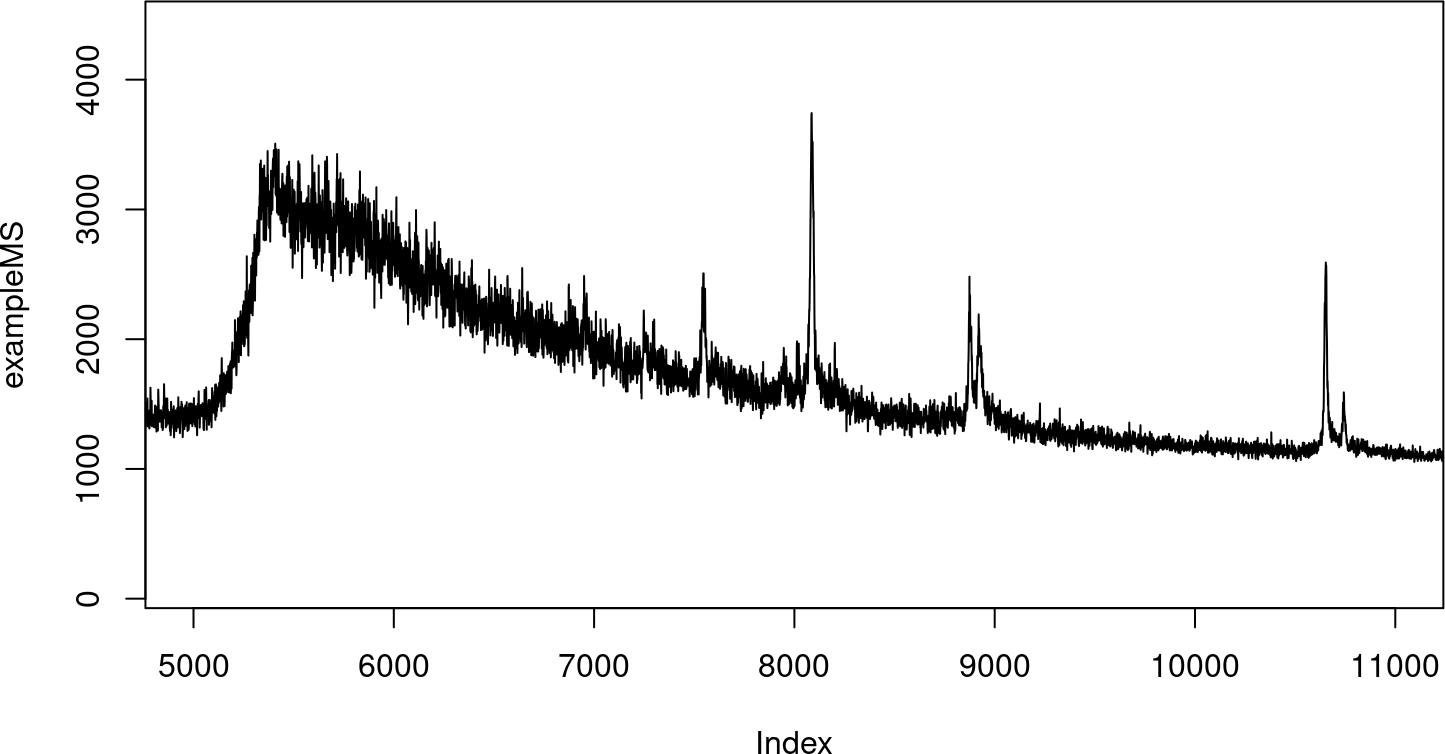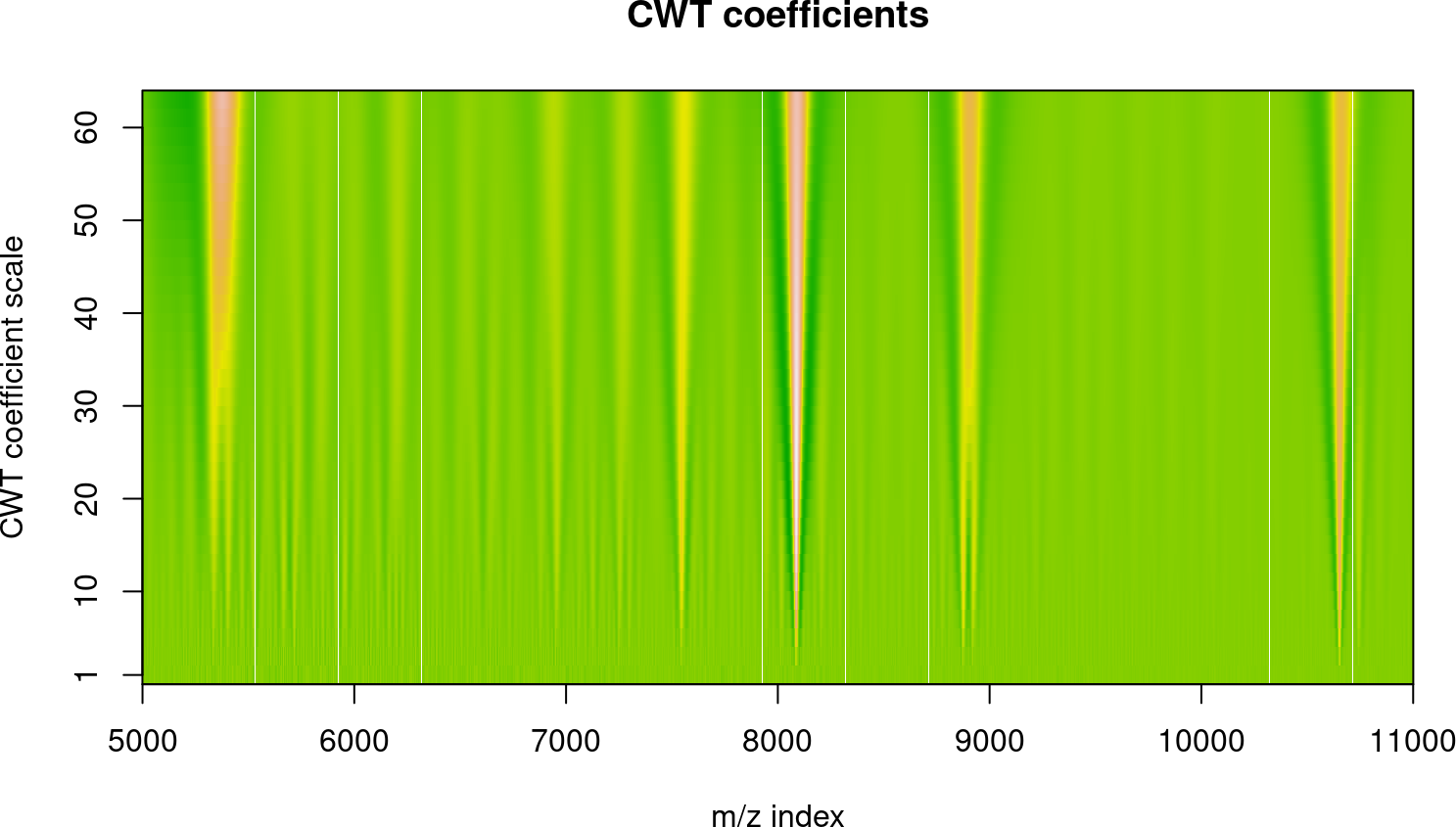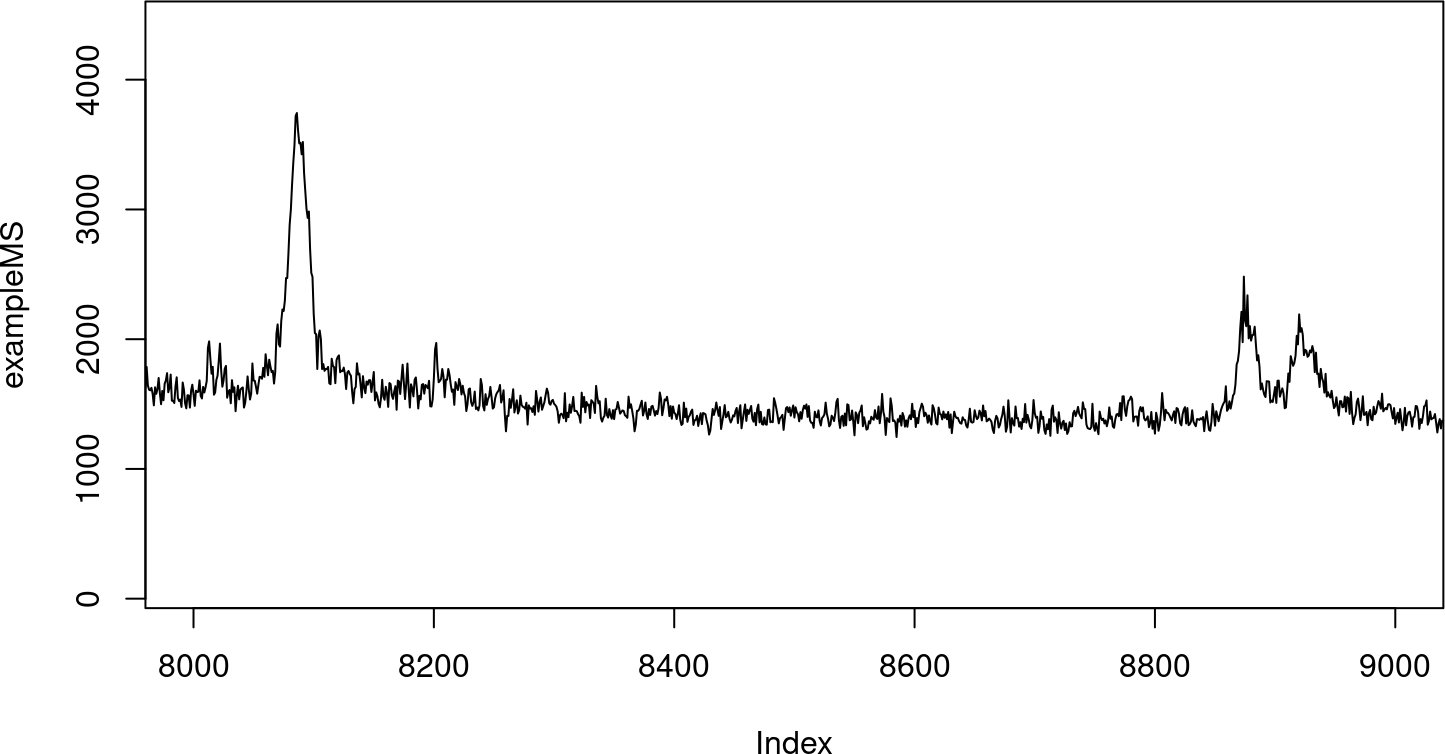# 1 Version Info

R version: R Under development (unstable) (2023-10-22 r85388)
Bioconductor version: 3.19
Package version: 1.69.0

# 2 Overview of MassSpecWavelet

MassSpecWavelet R package is aimed to process Mass Spectrometry (MS) data mainly based on Wavelet Transforms. The current version supports the peak detection based on Continuous Wavelet Transform (CWT). The algorithms have been evaluated with low resolution mass spectra (SELDI and MALDI data), we believe some of the algorithms can also be applied to other kind of spectra.

If you use MassSpecWavelet, please consider citing our work:

Du P, Kibbe W, Lin S (2006). “Improved peak detection in mass spectrum by incorporating continuous wavelet transform-based pattern matching.” Bioinformatics, 22(17), 2059-2065. ISSN 1367-4803, doi:10.1093/bioinformatics/btl355, https://academic.oup.com/bioinformatics/article-pdf/22/17/2059/767773/btl355.pdf, https://doi.org/10.1093/bioinformatics/btl355.

# 3 Peak detection by using CWT-based pattern matching

Motivation: A major problem for current peak detection algorithms is that noise in Mass Spectrometry (MS) spectrum gives rise to a high rate of false positives. The false positive rate is especially problematic in detecting peaks with low amplitudes. Usually, various baseline correction algorithms and smoothing methods are applied before attempting peak detection. This approach is very sensitive to the amount of smoothing and aggressiveness of the baseline correction, which contribute to making peak detection results inconsistent between runs, instrumentation and analysis methods.

Results: Most peak detection algorithms simply identify peaks based on amplitude, ignoring the additional information present in the shape of the peaks in a spectrum. In our experience, ‘true’ peaks have characteristic shapes, and providing a shape-matching function that provides a ‘goodness of fit’ coefficient should provide a more robust peak identification method. Based on these observations, a Continuous Wavelet Transform (CWT)-based peak detection algorithm has been devised that identifies peaks with different scales and amplitudes. By transforming the spectrum into wavelet space, the pattern-matching problem is simplified and additionally provides a powerful technique for identifying and separating signal from spike noise and colored noise. This transformation, with the additional information provided by the 2-D CWT coefficients can greatly enhance the effective Signal-to-Noise Ratio (SNR). Furthermore, with this technique no baseline removal or peak smoothing preprocessing steps are required before peak detection, and this improves the robustness of peak detection under a variety of conditions. The algorithm was evaluated with real MS spectra with known polypeptide positions. Comparisons with two other popular algorithms were performed. The results show the CWT-based algorithm can identify both strong and weak peaks while keeping false positive rate low.

## 3.1 Continuous wavelet transform with Mexican Hat wavelet

``data(exampleMS)``
``````plotRange <- c(5000, 11000)
plot(exampleMS, xlim = plotRange, type = "l")``````Continuous wavelet transform with Mexican Hat wavelet. The 2-D CWT coefficients image of MS spectrum in [5000, 11000] is shown in Figure 1

``````scales <- seq(1, 64, 2)
wCoefs <- cwt(exampleMS, scales = scales, wavelet = "mexh")``````
``````## Plot the 2-D CWT coefficients as image (It may take a while!)
xTickInterval <- 1000
plotRange <- c(5000, 11000)
image(
plotRange:plotRange,
scales,
wCoefs[plotRange:plotRange,],
col=terrain.colors(256),
axes=FALSE,
xlab='m/z index',
ylab='CWT coefficient scale',
main='CWT coefficients'
)
axis(1, at=seq(plotRange, plotRange, by=xTickInterval))
axis(2, at=c(1, seq(10, 64, by=10)))
box()``````Figure 1: 2-D CWT coefficient image

The smallest scales can be used for noise estimation, smaller scales are then useful for peaks with smaller width. Larger scales can detect wider peaks, at the expense of merging narrower peaks together.

``plot(exampleMS, xlim = c(8000, 9000), type = "l")````````matplot(
wCoefs[,ncol(wCoefs):1],
type = "l",
col = rev(rainbow(max(scales), start = 0.7, end = 0.1, alpha = 0.5)[scales]),
lty = 1,
xlim = c(8000, 9000),
xlab = "m/z index",
ylab = "CWT coefficients"
)
legend(
x = "topright",
legend = sprintf("scales = %d", scales[seq(1, length(scales), length.out = 4)]),
lty = 1,
col = rainbow(max(scales), start = 0.7, end = 0.1)[scales[seq(1, length(scales), length.out = 4)]]
)``````